# Use the stiffness method to determine the horizontal and vertical components of the displacement at Joints...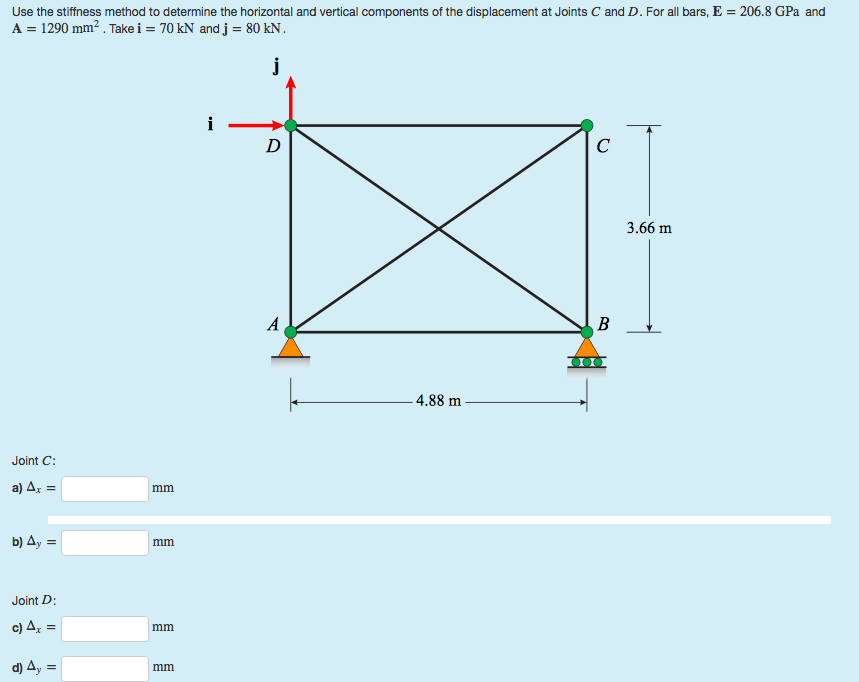Use the stiffness method to determine the horizontal and vertical components of the displacement at Joints C and D. For all bars, E = 206.8 GPa and A = 1290 mm². Take i = 70 kN and j = 80 kN. 3.66 m 4.88 m Joint C: a) Az = b) 4y = Joint D: c) 4, = d) 4, =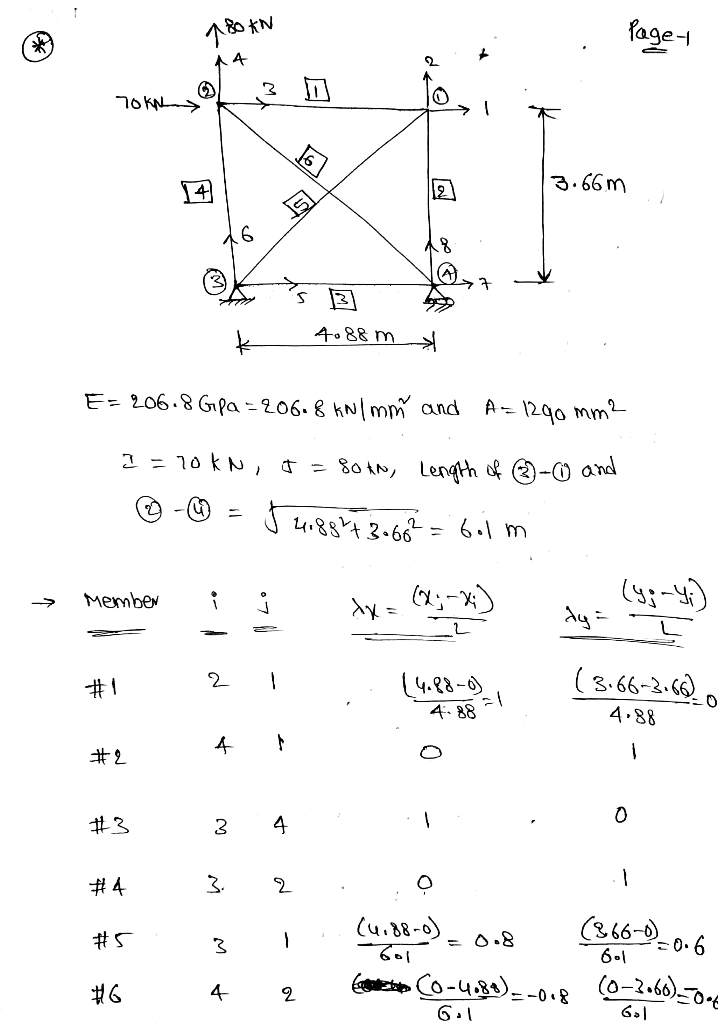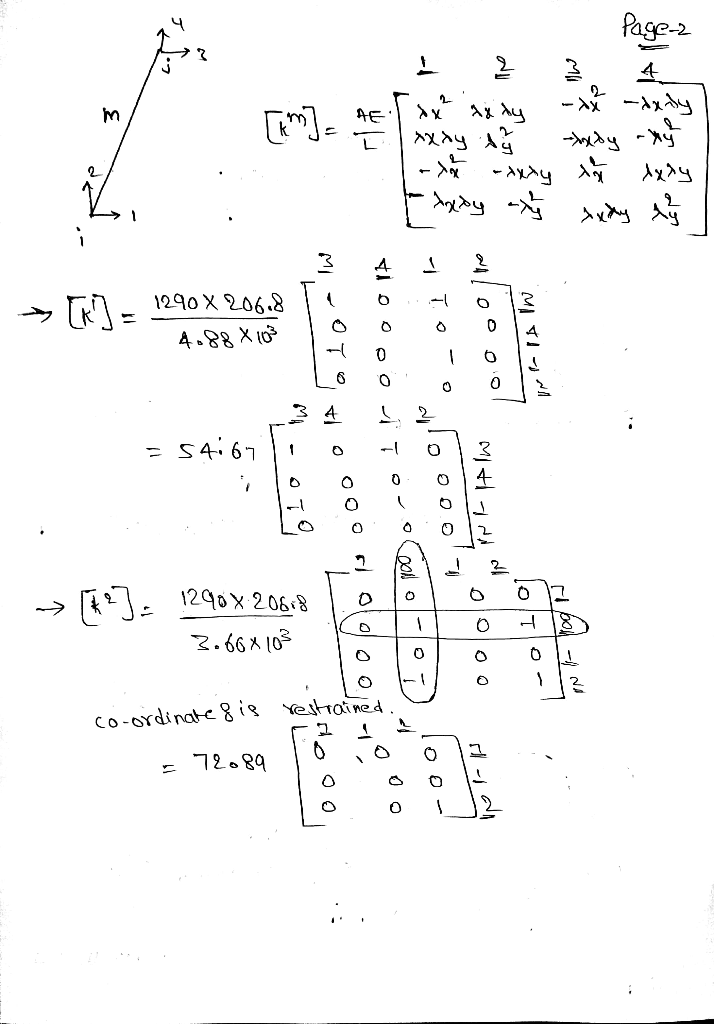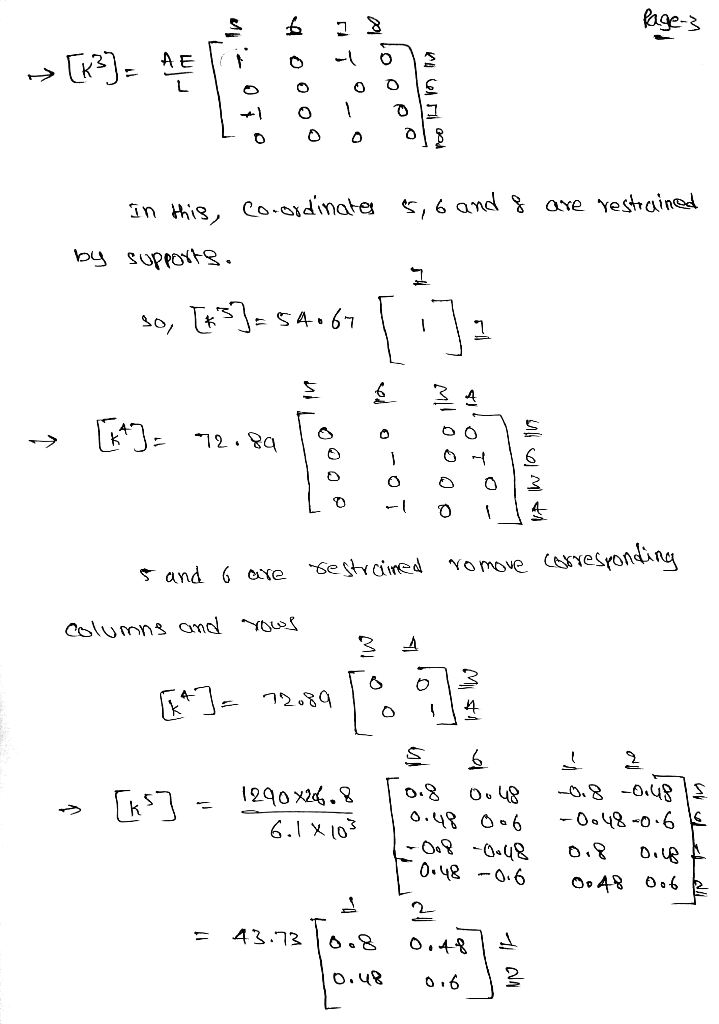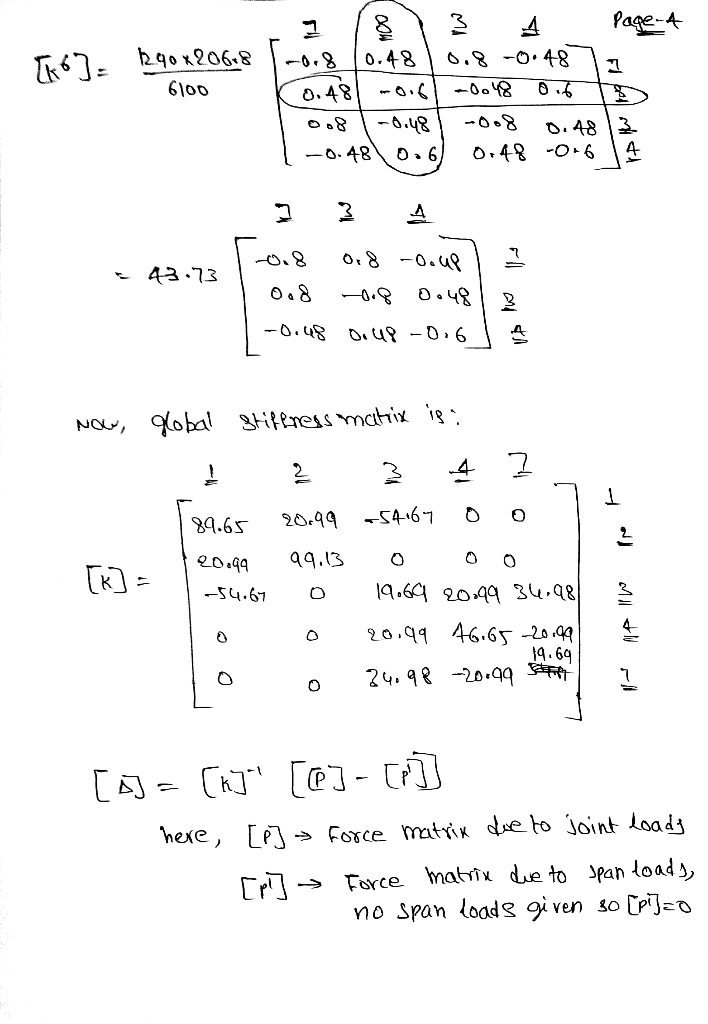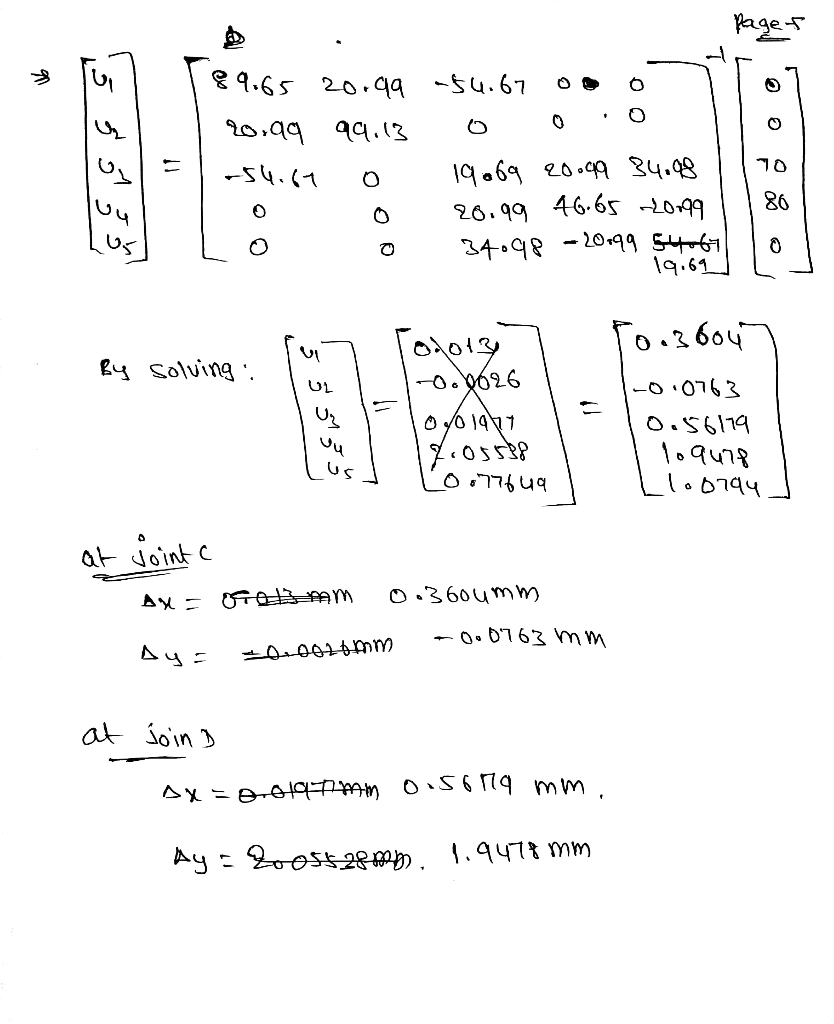#### Earn Coin

Coins can be redeemed for fabulous gifts.

Similar Homework Help Questions
• ### Week 7. Question 1: Use the stiffness method to determine the horizontal and vertical displacements at...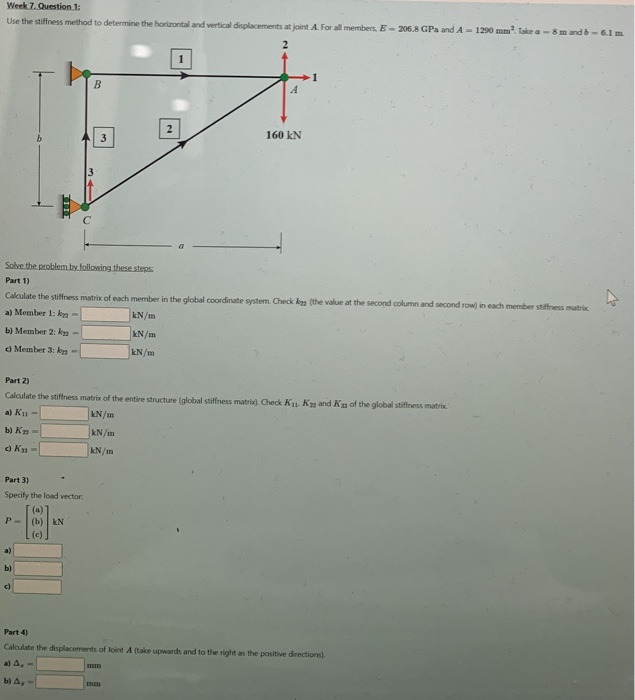Week 7. Question 1: Use the stiffness method to determine the horizontal and vertical displacements at joint A. For all members, E-206.8 GPa and A - 1290 mm? Take a - 8 mandb-6.1 m B 2 انها 160 kN Solve the problem by following these steps Part 1) Calculate the stiffness matrix of each member in the global coordinate system. Check kna (the value at the second column and second row) in each member stiffness matrix a) Member 1: ky...

• ### 2. Determine the vertical displacement at joint B and horizontal displacement at joint D using Castigliano's...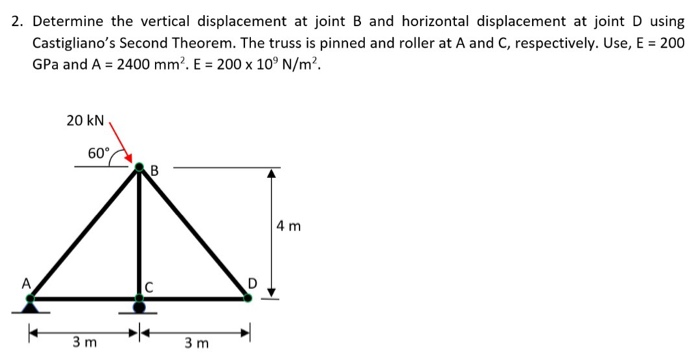2. Determine the vertical displacement at joint B and horizontal displacement at joint D using Castigliano's Second Theorem. The truss is pinned and roller at A and C, respectively. Use, E = 200 GPa and A = 2400 mm. E = 200 x 10°N/m². 20 KN 60 CAB 4 m 3m 3m

• ### Q3: Determine the vertical and horizontal displacement of joint A for the truss shown in Fig....Q3: Determine the vertical and horizontal displacement of joint A for the truss shown in Fig. (3). each bar is made of steel and has the cross-sectional area of 400mm Take E = 200 GPa Use the method of virtual work. E D 2 m |в -1.5 m -1.5 m 20 KN 40 KN Fig. (3)

• ### Q3: Determine the vertical and horizontal displacement of joint A for the truss shown in Fig....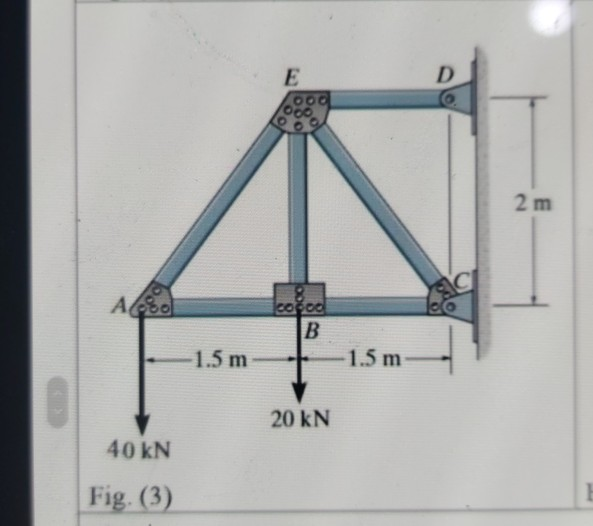Q3: Determine the vertical and horizontal displacement of joint A for the truss shown in Fig. (3), each bar is made of steel and has the cross-sectional area of 400mm?. Take E = 200 GPa. Use the method of virtual work. E D 00. 2 m СІ Ao cocoa B 1.5 m 1.5 m 20 KN 40 kN Fig. (3)

• ### Castigliano’s Theorem Determine the horizontal and vertical displacement at point E (in millimeters). E = 200...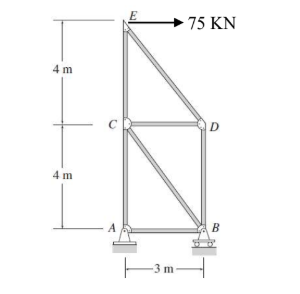Castigliano’s Theorem Determine the horizontal and vertical displacement at point E (in millimeters). E = 200 GPa. All the bars have an area of 5000 mm2 75 KN 4 m 4 m ITm

• ### tatically determinate or indeterminate frame analysis by the stiffness method (45 marks) a) Determine the stiffine...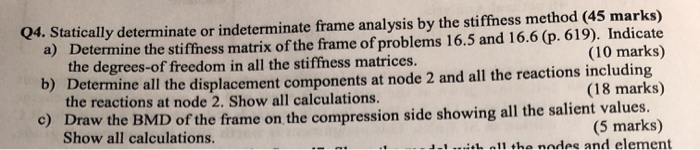tatically determinate or indeterminate frame analysis by the stiffness method (45 marks) a) Determine the stiffiness matrix of the frame of problems 16.5 and 16.6 (p. 619). Indicate the degrees-of freedom in all the stiffness matrices. b) D Q4. S (10 marks) etermine all the displacement components at node 2 and all the reactions including the reactions at node 2. Show all calculations. c) (18 marks) of the frame on the compression side showing all the salient values (5 marks)...

• ### i need the answer quickly fast as you can Q3: Determine the vertical and horizontal displacement...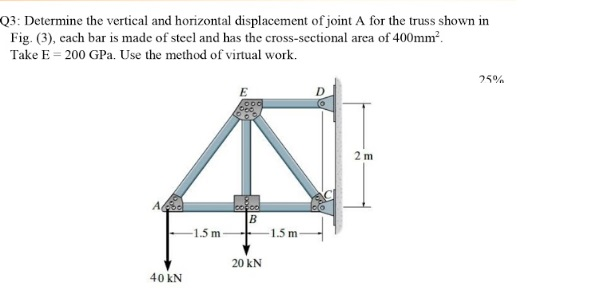i need the answer quickly fast as you can Q3: Determine the vertical and horizontal displacement of joint A for the truss shown in Fig. (3), cach bar is made of steel and has the cross-sectional area of 400mm? Take E = 200 GPa. Use the method of virtual work. 25% E 2 m А. cool B 1.5 m -1.5 m 20 KN 40 KN

• ### Use stiffness method with matrixes. *14-8. Determine the vertical displacement of join and the support reactions....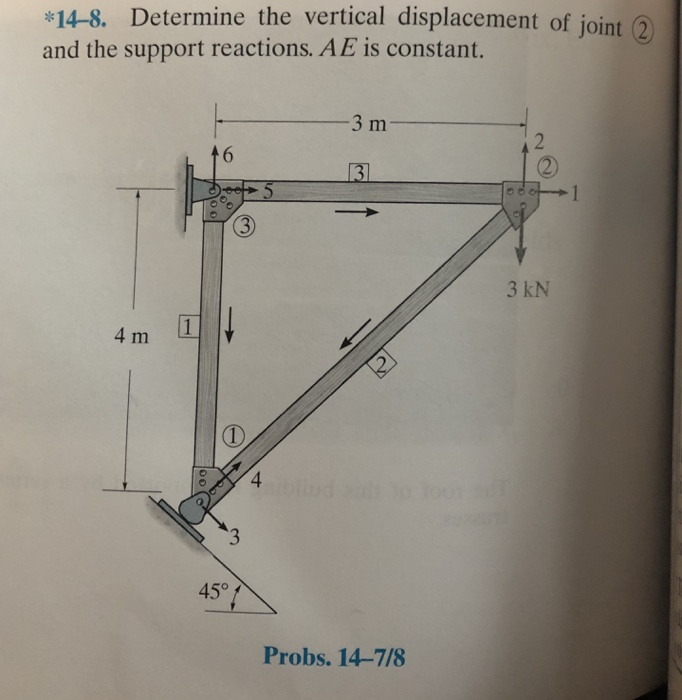Use stiffness method with matrixes. *14-8. Determine the vertical displacement of join and the support reactions. AE is constant. 3 m 3 kN 4 m 1 45° Probs. 14-7/8

• ### Solve It fast Please Q3: Determine the vertical and horizontal displacement of joint A for the...Solve It fast Please Q3: Determine the vertical and horizontal displacement of joint A for the truss shown in Fig. (3). cach bar is made of steel and has the cross-sectional area of 400mmº. Take E = 200 GP. Use the method of virtual work. 25% E OO D 2 m AAS ered B -1.5 m -1.5 m 20 KN 40 KN Fig. (3)

• ### The horizontal displacement of the joint where the forces are applied is: a) 0.015 mm b)...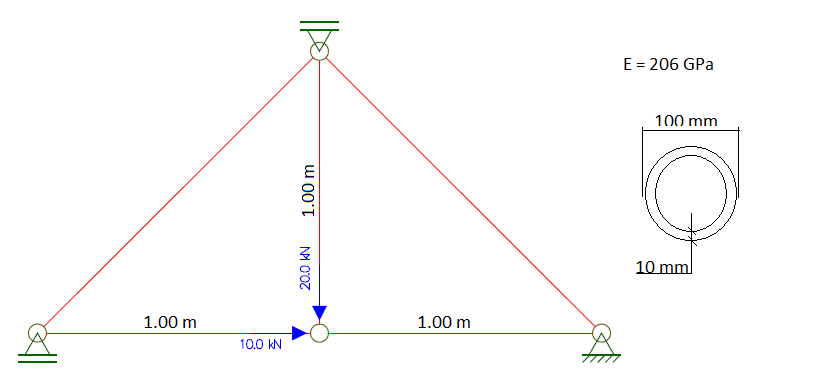The horizontal displacement of the joint where the forces are applied is: a) 0.015 mm b) 0.034 mm c) 0.001 mm d) 0.007 mm e) 0.068 mm E 206 GPa 1.00 m 1.00 m 10.0 kN